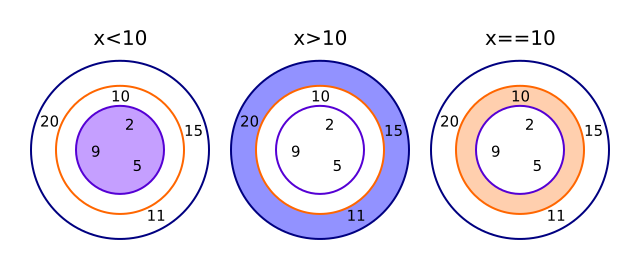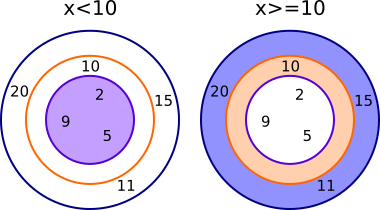# Conditions and programming logic

Martin McBride, 2017-01-17
Tags condition relational operator
Categories logic programming logic

A condition is an expression which is either true or false, for example:

x > 10


Depending on the value of x, this expression is either true or false - x is either greater than 10, or it is not.

We call > (greater than) a relational operator.

## The 3 basic relational operators

The 3 basic relational operators are less than, greater than and equals.

In this diagram, the inner circle contains values which are less than 10, the middle circle contains the value 10, and the outer circle contains values which are greater than 10:We can shade the areas to represent different conditions:The inner circle represents the condition x is less than 10, usually written as x < 10.

The outer circle represents the condition x is greater than 10, usually written as x > 10.

The middle circle represents the condition x equals 10, usually written as x == 10. Notice that we use a double equals sign for equality, because writing x = 10 means "set x equal to 10".

Most languages use these symbols, although some older languages are different, for example they might use x LT 10 for less than.

## More relational operators

There are 3 more relational operators, which can be found by shading 2 circles:The outer 2 circles represent the condition that x is greater than or equal to 10. This is usually written as x >= 10.

The inner 2 circles represent the condition that x is less than or equal to 10. This is usually written as x <= 10.

What if we shade the outer circle and the inner circle, but not the middle circle? This represents the case where x is either greater than 10, or less than 10. In other words, it means that x is not equal to 10. This is usually written as x != 10.

As an example, suppose we have a computer game which is so scary that you have to be at least 10 to play it. The following code wouldn't work:

if age > 10
print("You can play the game")


Do you see the problem? Someone who was actually 10, who should be able to play the game, would fail the test because their age isn't greater than 10. Here is the correct test:

if age >= 10
print("You can play the game")


## Opposites

Some of these conditions are opposites of each other.

What is the opposite of less than? At first, you might think it is greater than, but that isn't quite right. In fact, the opposite of less than is greater than or equal.

Look at this code:

if not age < 10
print("You can play the game")


This does the same thing as the previous (working) code. If your age is not less than 10, that is exactly the same as saying your age is greater than or equal to 10. We can also see that from the shaded rings, the circle which is shaded in the < case is the only circle which isn't shaded in the >= case:Here are the 3 opposites:

• < is opposite to >=
• > is opposite to <=
• == is opposite to !=